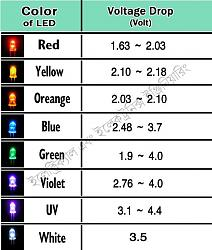Free 186 More Best Homemade Tools eBook:

# Thread: Calculate LED current limiting resistor

1. ## Calculate LED current limiting resistor

LEDs are used often enough in home projects that it's worth a short tutorial on determining the proper resistor to be used with one.

The breakdown voltage of an LED (Vb) varies with color. This chart shows ranges of that voltage...A typical supply current is I=20 ma. The required resistor can then be calculated from Ohm's law (R = V/I)

R = (Vs - Vb)/0.02

here Vs is the power supply voltage.

An example will help. Suppose we have a red LED we wish to power with a 12 volt battery. From the figure, the average Vb for a red LED is 1.9 volts.
Then:

R = (12-1.9) / 0.02 = 10.1 * 50 = 505 ohms

The standard series of resistors looks like..

1 1.2  1.5  1.8  2.2  2.7  3.3  3.9  4.7  5.6  6.8  8.2  10

All of these values can be multiplied by powers of ten (1.2  12  120 - 1200, etc.).

So the closest we can come to 505 is a 470 ohm resistor.

The resistor color code is

0 black
1 brown
2 red
3 orange
4 yellow
5 green
6 blue
7 violet
8 gray
9 white

The third color band indicates the power of ten multiplier.

so a 470 ohm resistor will have yellow-violet-brown bands.

Most LEDs will have a flat area on the base to indicate the cathode (negative) terminal. Sometimes, the cathode lead is made shorter to indicate which it is.Reply With Quote

2. ## The Following 4 Users Say Thank You to mklotz For This Useful Post:

DIYer (Dec 20, 2021), mr_modify1 (Dec 23, 2021), rebuilder1954 (Dec 22, 2021), sparky42 (Dec 22, 2021)

3. Thanks mklotz! We've added your LED Limiting Resistor Calculation to our Electrical category,Reply With Quote

4. The wattage, W, being dissipated in the resistor is given by...

W = I²R = 0.02² * 470 = 4E-4 * 470 = 0.188 watts

The common standard power ratings of resistors are 0.25W, 0.5W, 1W, 2W, 5W, and 25W so even the smallest size can be used in this application.Reply With Quote

5. I've learned the colour coding in the following.
Bad Boys Rape Our Young Girls But Violet Gives Willingly.

Bad = Black - 1 Ohm = 1 Ohm
Boys = Brown - 10 Ohm = 10 Ohm
Rape = Red - 100 Ohm = 100 Ohm
Our = Orange - 1000 Ohm = 1K Ohm
Young = Yellow - 10000 Ohm = 10K Ohm
Girls = Green - 100000 Ohm = 100K Ohm
But = Blue - 1000000 Ohm = 1M Ohm
Violet = Violet - 10000000 Ohm = 10M Ohm
Give = Grey - 100000000 Ohm = 100M Ohm
Willingly = White - 1000000000 Ohm = 1G Ohm

Just sayingReply With Quote

6.Originally Posted by SaaglemI've learned the colour coding in the following.
Bad Boys Rape Our Young Girls But Violet Gives Willingly.

Bad = Black - 1 Ohm = 1 Ohm
Boys = Brown - 10 Ohm = 10 Ohm
Rape = Red - 100 Ohm = 100 Ohm
Our = Orange - 1000 Ohm = 1K Ohm
Young = Yellow - 10000 Ohm = 10K Ohm
Girls = Green - 100000 Ohm = 100K Ohm
But = Blue - 1000000 Ohm = 1M Ohm
Violet = Violet - 10000000 Ohm = 10M Ohm
Give = Grey - 100000000 Ohm = 100M Ohm
Willingly = White - 1000000000 Ohm = 1G Ohm

Just saying
Yes, that and its less PC variants have been a mnemonic for the code for a long time.

However, each color denotes a digit, not a resistance as you have indicated. It's the combination of three digits that denote the resistance.Reply With Quote

7. [QUOTE=mklotz;194134]LEDs are used often enough in home projects that it's worth a short tutorial on determining the proper resistor to be used with one.

The breakdown voltage of an LED (Vb) varies with color. This chart shows ranges of that voltage...

Marv,

A bit of poking around for infrared LEDs (which I occasionally use in isolation applications) yields Vb around 1.9v.

BobReply With Quote

8. Your right.... my mistake. That ohm should not have been there!Reply With Quote

9.Reply With Quote